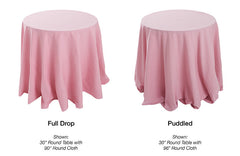Monday-Friday 10:00-6:00 Saturday 10:00-5:00 Closed Sunday

# How To Figure Out Your Tablecloth Sizes

tablecloth tutorial wedding table linens

Shopping for wedding tablecloths online can be very overwhelming, especially when your wedding is rapidly approaching. Knowing the correct wedding tablecloth sizes for the tables at your venue is of utmost importance. Luckily, it is very easy to order the perfect tablecloths for your event tables. All you need to know to properly size your wedding tablecloths is the size of the tables you are planning to cover. Also, you will need to take into account your own aesthetic taste. Do you like a half, full, or puddle drop?In general, a full drop is regarded as a very formal look while the half drop is considered more casual. A puddle drop can be amazingly elegant, depending on the setting and mood you are trying to convey. Now that you know what look appeals to you, you can take a look at the specific measurements for tablecloth sizes based on table sizes. All of the following measurements assume that round, square, and rectangular tables measure 30 inches in height. Want to size your tablecloths the easy way? Try our free tablecloth sizing tool.

Round Tables
Half Drop:
30 inch round table (seats 2-4) needs a 51 inch or 70 inch round tablecloth
36 inch round table (seats 2-4) needs a 70 inch round tablecloth
42 inch round table (seats 4-5) needs a 70 inch round tablecloth
48 inch round table (seats 4-5) needs a 76 inch round tablecloth
54 inch round table (seats 6-8) needs a 90 inch round tablecloth
60 inch round table (seats 8-10) needs a 90 inch round tablecloth
66 inch round table (seats 9-11) needs a 90 inch round tablecloth
72 inch round table (seats 10-12) needs a 108 inch round tablecloth

Full Drop:
30 inch round table (seats 2-4) needs a 90 inch round tablecloth
36 inch round table (seats 2-4) needs a 90 inch round tablecloth
42 inch round table (seats 4-5) needs a 108 inch round tablecloth
48 inch round table (seats 4-5) needs a 108 inch round tablecloth
54 inch round table (seats 6-8) needs a 120 inch round tablecloth
60 inch round table (seats 8-10) needs a 120 inch round tablecloth
66 inch round table (seats 9-11) needs a 120 inch round tablecloth
72 inch round table (seats 10-12) needs a 132 inch round tablecloth

Puddle Drop:
30 inch round table (seats 2-4) needs a 108 inch round tablecloth
36 inch round table (seats 2-4) needs a 120 inch round tablecloth
42 inch round table (seats 4-5) needs a 120 inch round tablecloth
48 inch round table (seats 4-5) needs a 132 inch round tablecloth
54 inch round table (seats 6-8) needs a 132 inch round tablecloth

To calculate the drop of your round wedding tablecloth, subtract the table diameter from the linen diameter and then divide by two. For example, if you have a 72 inch table and a 132 inch wedding tablecloth, subtract 72 from 132 (132-72) to get 60. Then divide 60 by 2 for a full drop of 30 inch.

Square Tables
Half Drop:
30 inch square table (seats 4) needs a 60 inch square tablecloth
36 inch square table (seats 4) needs a 60 inch square tablecloth
42 inch square table (seats 8) needs a 72 inch square tablecloth
48 inch square table (seats 8) needs a 85 inch square tablecloth
54 inch square table (seats 8) needs a 85 inch square tablecloth
60 inch square table (seats 8-12) needs a 85 inch square tablecloth

Full Drop:
30 inch square table (seats 4) needs a 85 inch square tablecloth
36 inch square table (seats 4) needs a 85 inch square tablecloth

A square table’s measurement is taken from one side, and the drop can be calculated with a simple formula: subtract the table length from the linen length, then divide by two. For instance, if you have a 36 x 36 inch table and an 85 x 85 inch tablecloth, you subtract 85 from 36 (85-36) to get 49. Then divide 49 by 2 for a final drop of 24.5 inch. That is nearly 30 inches, so this drop could be considered a full drop as long as the table is 30 inches in height.

Rectangular or Oblong Tables
Half Drop:
6 ft. rectangular table (seats 6) needs a 60 x 102 inch rectangular tablecloth
8 ft. rectangular table (seats 8) needs a 60 x 126 inch rectangular tablecloth

Full Drop:
6 ft. rectangular table (seats 6) needs a 90 x 132 inch rectangular tablecloth
8 ft. rectangular table (seats 8) needs a 90 x 156 inch rectangular tablecloth

Puddle Drop:
6 ft rectangular table (seats 6) needs a 90 x 156 inch rectangular tablecloth

Figuring out the drop of a rectangular or oblong table is very easy, too. Subtract the table length from the linen length and the table width from the linen width, and then divide both differences by two. That means if you have a 4ft. (30 x 48 in.) rectangular table with a 70 x 120 inch tablecloth, start by subtracting 30 from 70 (70-30=40) and 48 from 120 (120-48=72). Then you divide 40 by 2 (40/2=20) and 72 by 2 (72/2=36) which means your tablecloth will drop 20 inches widthwise and 36 inches lengthwise. Once again, this formula assumes that your table measures 30 inches height wise.

We hope you found this wedding tablecloth information useful. If you have questions, feel free to leave us a comment below. Please share if you found this a useful resource!